Electron. J. Diff. Eqns., Vol. 2007(2007), No. 144, pp. 1-8.

### Non-symmetric elliptic operators on bounded Lipschitz domains in the plane David J. Rule

Abstract:
We consider divergence form elliptic operators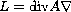in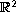with a coefficient matrix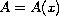of bounded measurable functions independent of the-direction. The aim of this note is to demonstrate how the proof of the main theorem in  can be modified to bounded Lipschitz domains. The original theorem states that the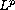Neumann and regularity problems are solvable for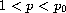for some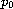in domains of the form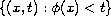, where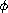is a Lipschitz function. The exponentdepends only on the ellipticity constants and the Lipschitz constant of. The principal modification of the argument for the original result is to prove the boundedness of the layer potentials on domains of the form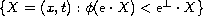, for a fixed unit vectorand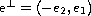. This is proved in  only in the case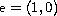. A simple localisation argument then completes the proof.

Submitted October 10, 2007. Published October 30, 2007.
Math Subject Classifications: 35J25, 31A25.
Key Words: T(b) Theorem; layer potentials;Neumann problem;regularity problem; non-symmetric elliptic equations.

Show me the PDF file (236 KB), TEX file, and other files for this article.David J. Rule School of Mathematics and Maxwell Institute for Mathematical Sciences The University of Edinburghm James Clerk Maxwell Building The King's Buildings, Mayfield Road Edinburgh, EH9 3JZ, UK email: rule@uchicago.edu